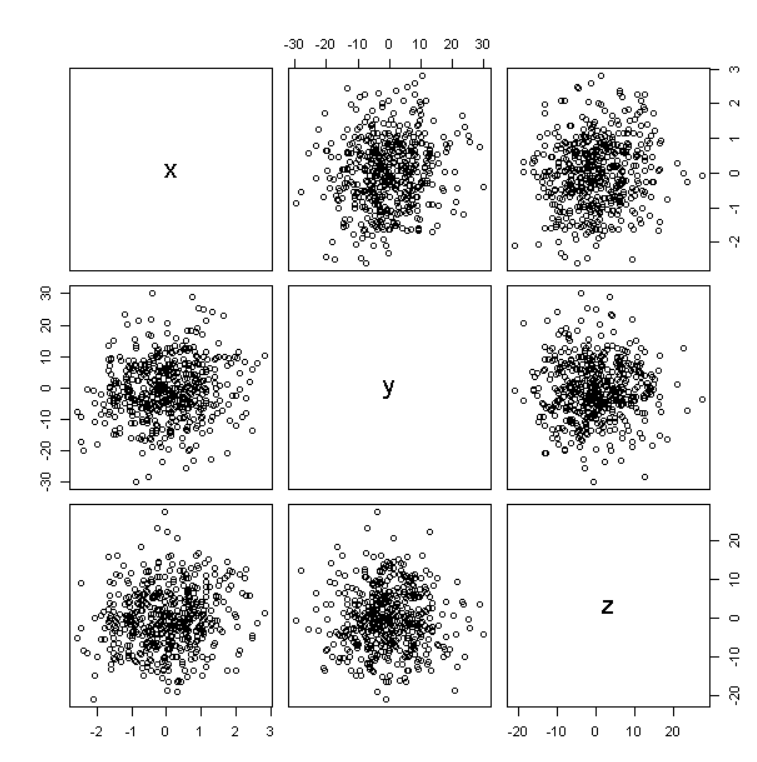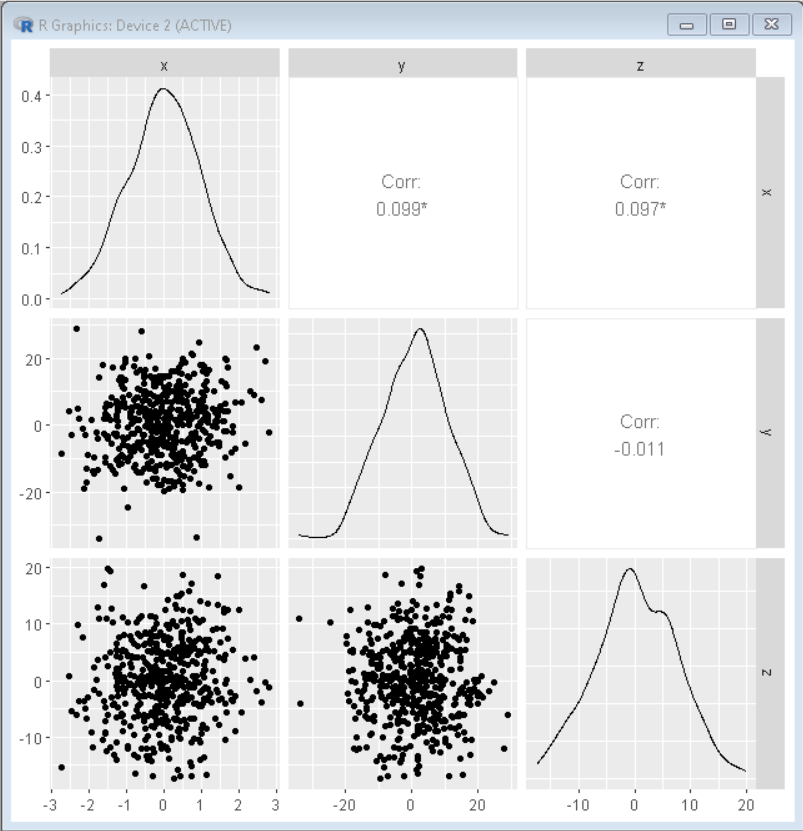# How to Create and Interpret Pairs Plots in R?

• Last Updated : 27 Jan, 2022

In this article, we will discuss how to create and interpret Pair Plots in the R Language.

The Pair Plot helps us to visualize the distribution of single variables as well as relationships between two variables. They are a great method to identify trends between variables for follow-up analysis. Pair plots are essentially multipanel scatter plots where every different panel contains a scatter plot between a pair of variables.

## Method 1: Create Pair Plots in Base R

To create a Pair Plot in the R Language, we use the pairs() function. The pairs function is provided in R Language by default and it produces a matrix of scatterplots. The pairs() function takes the data frame as an argument and returns a matrix of scatter plots between each pair of variables in the data frame.

Syntax: pairs( df )

Parameter:

• df: determines the data frame used for plotting to scatter plot.

Example:

Here, is a basic Pair Plot in Base R.

## R

 `# create sample_data ` `x <- ``rnorm``(500) ` `y <- x + ``rnorm``(500, 0, 10) ` `z <- x - ``rnorm``(500, 0, 7) ` `  `  `sample_data <- ``data.frame``(x, y, z) ` ` `  `#create pairs plot  ` `pairs``( sample_data )`

Output:Here, in the above pair plot, diagonal boxes show the name of variables x, y, and z. All other boxes display a scatterplot between each pairwise combination of variables. For example, the second box shows a scatterplot between x and y whereas the third box shows a scatter plot between x and z.

The problem with this pair plot is that this doesn’t give us any statistical information about variables and there are only three distinguished scatter plots out of six in the above figure as x-z and z-x plot is same, y-x and x-y plot is same, and y-z and z-y plot is same. So, there is a wastage of space as well as the absence of relational data. To solve this we use the ggplot2 package.

## Method 2: Create Pair Plots Using ggplot2 and ggally

To create a pair plot using the ggplot2 package, we use the ggpairs() function of the ggally package. The ggally package is an extension of the ggplot2 package which extends the ggplot2 package by adding several functions to reduce the complexity of combining the geoms with transformed data. The ggpairs() function makes a matrix of plots with a given data set. It produces scatter plots for each pair of variables, density plots for each variable, and also shows the Pearson Correlation Coefficients of each pair of variables.

Syntax:

ggpairs( df )

Parameter:

• df: determines the data frame used for plotting to scatter plot.

Example:

Here, is a basic Pair Plot using the ggplot2 and ggally package library.

## R

 `# load libraries ggplot2 and ggally ` `library``(ggplot2) ` `library``(GGally) ` ` `  `# create sample_data ` `x <- ``rnorm``(500) ` `y <- x + ``rnorm``(500, 0, 10) ` `z <- x - ``rnorm``(500, 0, 7) ` `  `  `sample_data <- ``data.frame``(x, y, z) ` ` `  `# create pairs plot ` `ggpairs``( sample_data )`

Output:Here, in the above pair plot, the variable names are displayed on the outer edges of the matrix as x, y, and z. The boxes along the diagonals display the density plot for each variable whereas the boxes in the lower-left corner display the scatterplot between each -pair of variables. The boxes in the upper right corner display the Pearson correlation coefficient between each variable.

The Pearson correlation gives us the measure of the linear relationship between two variables. It has a value between -1 to 1, where a value of -1 signifies a total negative linear correlation, 0 signifies no correlation, and + 1 signifies a total positive correlation.

The pair plots made by using the ggplot2 package are better because they give more visual information with no repetition of the same plot. They also give us the Pearson correlation coefficient which helps us in understanding the relationship between those variables.

My Personal Notes arrow_drop_up
Recommended Articles
Page :